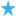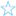Windows has included a calculator for many years now, and for basic day to day calculations it functions well. For more advanced calculation, you have the option of switching to scientific mode, but there are circumstances in which even this can be somewhat underpowered.

Microsoft Mathematics is a surprisingly power calculator that in many ways functions like a traditional handheld calculator. It features a graphing option that can be used to visualise equations in mathematics and science.

This is much more than a basic scientific calculator - Microsoft Mathematics also includes a unit conversion utility and can be used to calculate calculus, algebra and trigonometry. When working with equations, the option to generate 2- and 3D graphs is immensely useful.

The program includes a step-by-step equation solver that will not only help you to break down an equation or problem and work through it gradually, but also to gain a better understanding of the principles as work. With a packed functions and equations library as well as a triangle solver, this is a great addition to any students set of tools.

## VerdictA great improvement on Windows' built in calculator that will be genuinely useful to students.